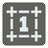The Hamming Distance
Elementary+
JA UK RU English FR HU

The Hamming distance between two binary integers is the number of bit positions that differs (read more about the Hamming distance on Wikipedia). For example:

```    117 = 0 1 1 1 0 1 0 1
17 = 0 0 0 1 0 0 0 1
H = 0+1+1+0+0+1+0+0 = 3

```

You are given two positive numbers (N, M) in decimal form. You should calculate the Hamming distance between these two numbers in binary form.

Input: Two arguments as integers.

Output: The Hamming distance as an integer.

Example:

```checkio(117, 17) == 3
checkio(1, 2) == 2
checkio(16, 15) == 5
```

How it is used: This is a basis for Hamming code and other linear error-correcting programs. The Hamming distance is used in systematics as a measure of genetic distance. On a grid (ie: a chessboard,) the Hamming distance is the minimum number of moves it would take a rook to move from one cell to the other.

Precondition:
0 < n < 106
0 < m < 106